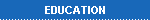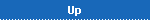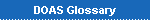ResearchNavigate## What is DOAS?

The Differential Optical Absorption Spectroscopy (DOAS) is a method to determine the concentrations and total amounts of atmospheric trace gases from remote sensing measurements of light in the UV, visible, and NIR spectral range. The basic principle used in DOAS is absorption spectroscopy. Light travelling through the atmosphere is partly absorbed by trace constituents along the way following Lambert Beer's law of absorption:The intensity I(λ, s) at the end of a light path depends on

• the absorption strength of the species of interest at the wavelength λ observed σ(λ)
• the initial intensity I0
• the length of the light path s
• the total amount of the absorber along the light path which for a constant concentration of the absorber ρ is just the product of concentration and path length: ρs

In the following, we will have a closer look at all these quantities for atmospheric DOAS measurements.

## The absorption Cross-Section

The absorption cross-section of a trace gas its ability to absorb incoming photons as a function of wavelength and is given in units of cm2 per molecule. It can be determined in the lab and therefore here is considered as well known. It usually depends on temperature and often also on pressure which has to be taken into account in the data analysis. As an example, the absorption cross-section of NO2 is shown, both over the complete wavelength range covered by the GOME instrument and in the region often used for NO2 retrieval (inset). The latter is already high pass filtered to highlight the differential structures which are used to separate absorption by trace species and extinction by scattering (see below).## The initial Intensity

In a laboratory experiment, the initial intensity of the lamp can be monitored and determined with high accuracy. Often it is determined by removing the absorbing species from the light path e.g. by filling a cell with nitrogen instead of NO2.

For atmospheric measurements, this is usually not an option, and other approaches have to be taken to remove the unknown initial intensity from the equation. There basically are two options:

1. Use of measurements at different light paths. If it is possible to take two measurements with the same initial intensity but at different light path lengths s, the dependence on the initial intensity cancels if one looks at the ratio of the two measurements:This approach is e.g. used in zenith-sky DOAS, where a measurement at low sun (long light path through the atmosphere) is analysed with a measurement taken at high sun (short light path). See also our MAXDOAS page for more on the measurement geometry.
2. Use of measurements at different wavelengths. In this approach, one exploits the fact that many molecules have structured spectra and light at different wavelengths experiences different absorption strengths. If the initial intensity does not vary with wavelength, one can look at the ratio of two measurements at different wavelengths to remove the dependence on I0:This approach is often used in long-path measurements which employ a lamp. It also is one reason to call this method differential as changes in absorption are used.

## The Light PathThe length of the light path is a simple geometric quantity in the lab. It also can be determined with high accuracy if a lamp (A) or direct sun or moon (B) light is used for atmospheric measurements. However, if scattered light is used as is the case for zenith-sky (C) or horizon DOAS observations and also for satellite nadir (D) and limb measurements, multiple paths through the atmosphere contribute with different weights. For these applications, the effective light path is determined using radiative transfer models such as SCIATRAN.

It is often useful to express the light path not in absolute units (such as km) but as an airmass factor which is defined as the ratio of the number of molecules per cm2 seen in a measurement (slant column density) relative to that expected for a single, vertical transect of the atmosphere (vertical column density):More on airmass factors and a tool to visualise satellite AMFs for different scenarios can be found on our airmass factor page.

## Dealing with multiple Absorbers

In the real atmosphere, multiple effects contribute to the overall attenuation of the light. In particular, aerosols and clouds scatter light and thereby reduce the intensity of the direct beam while increasing intensities measured in other directions. Also, there rarely is only one absorber relevant at a given wavelength and this also needs to be accounted for.

The solution to this problem lies in the use of measurements at several wavelengths. Each molecule has a characteristic absorption spectrum (its spectral fingerprint) and therefore, simultaneous measurements at different wavelengths should enable us to separate the contributions of the different absorbers. Basically, one uses many equations of the typeto describe the problem. Using more wavelengths λi facilitates determination of more unknowns ρj, but usually the number of wavelengths used is much larger than the number of absorbers to be retrieved.

## Accounting for Aerosols

What about aerosols? Fortunately, their extinction cross-sections can be approximated by power laws (λ-4 for Rayleight scattering and λ-1..0 for Mie scattering), the coefficients of which can be determined in the fit. The basic principle behind the separation of aerosol extinction and trace gas absorption is that the latter are identified using those parts of their absorption cross-sections that vary rapidly with wavelength. The more slowly varying parts of the absorption can not be separated from extinction by aerosols which is why the absorption cross-sections are often high pass filtered before use in a DOAS retrieval.

In addition to their effect on the spectral distribution of the intensity measured, aerosols can also have a significant impact on the light path of scattered light. This has to be modelled explicitly when computing the airmass factors for the light path correction.

 web master:  folkard@iup.physik.uni-bremen.de      Last Update: 25-05-18      Data Privacy